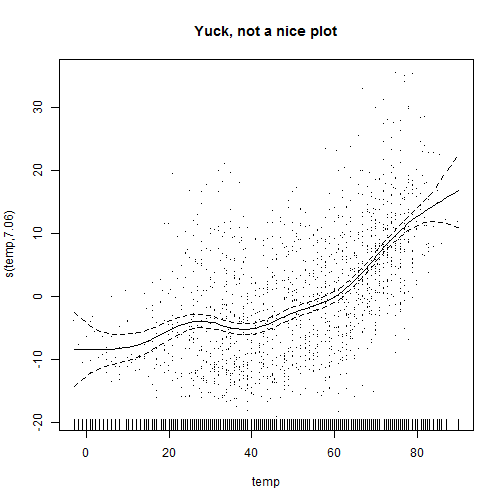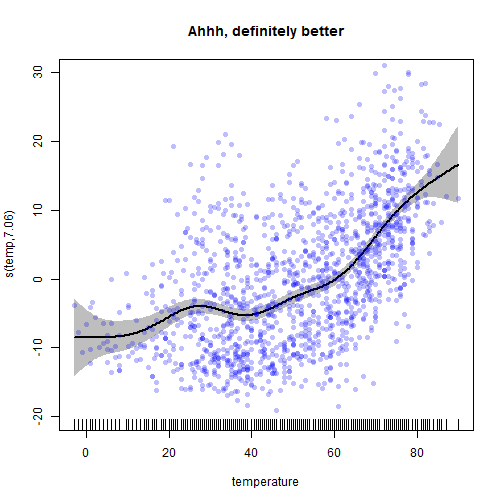# Recreate the GAM partial regression smooth plots from R package `mgcv` (with a little style)

We use the R library `mgcv` for modeling environmental data with generalized additive models (GAMs). It's a great library loaded with functionality but we often find that the default diagnostic plots are uninspiring. The partial residual plots, in particular, are functional but not pretty and the residuals are almost invisible.

This post would be much more useful if we created a clean and flexible R function and posted to GitHub but for now you'll need to make your own based on these code hints. We’re also the first to admit that yes, this is boring material for many. At the same time we’re assuming that there are a few people who are, like us, unhappy with the look of the default partial regression plots but need a little guidance on code.

Update 2014-09-17: Note that three different viewers of this post re-wrote the code using pipes (using the R packages `pipeR` or `magrittr`). If you want to see their code:

Here is @timelyportfolio’s version (using `pipeR`).
Here is @smbache’s version (using `magrittr`).
Here is @renkun_ken’s version (using `pipeR`).

### 1) The basic data set-up

``````library(ggplot2)
library(mgcv)
nmmaps\$date<-as.Date(nmmaps\$date)
nmmaps<-nmmaps[nmmaps\$date>as.Date("1996-12-31"),]
nmmaps\$year<-substring(nmmaps\$date,1,4)
``````

### 2) A simple GAM model – Temperature vs Ozone

For this example, we will keep the model simple – Gaussian data, a single predictor. We're modeling temperature against ozone in Chicago and we will output the default partial residual plot.

``````# model - temperature against ozone
thegam<-gam(o3~s(temp), data=nmmaps)
plot(thegam, residuals=TRUE, main="Yuck, not a nice plot")
``````Do you agree that this plot could be improved?

### 3) Recreate the partial residual plot

Here we add the smooth term, confidence intervals and partial residuals.

``````# create a sequence of temperature that spans your temperature
maxtemperature<-max(nmmaps\$temp)
mintemperature<-min(nmmaps\$temp)
temperature.seq<-seq(mintemperature, maxtemperature, length=300)
temperature.seq<-data.frame(temp=temperature.seq)

# predict only the temperature term (the sum of the
# term predictions and the intercept gives you the overall
# prediction)

preds<-predict(thegam, type="terms", newdata=temperature.seq,
se.fit=TRUE)

# set up the temperature, the fit and the upper and lower
# confidence interval

temperature<-temperature.seq\$temp
fit<-preds\$fit
fit.up95<-fit-1.96*preds\$se.fit
fit.low95<-fit+1.96*preds\$se.fit

# plot the temperature smooth but leave blank for
# now so that we can add the line on top of the polygon
plot(temperature, fit, type="n", lwd=3, xlim=c(-3,90), ylim=c(-20,30),
main="Ahhh, definitely better",
ylab=paste("s(temp,", round(sum(thegam\$edf[-1]),2), ")", sep=""))

# If you want confidence lines instead of a grey poly you can
# use this code
#lines(temperature, fit.up95, lty="dotted")
#lines(temperature, fit.low95, lty="dotted")

# For the confidence grey polygon
polygon(c(temperature, rev(temperature)),
c(fit.low95,rev(fit.up95)), col="grey",
border=NA)

lines(temperature, fit,  lwd=2)
``````

In a final step we want to add the partial residuals themselves. The partial residuals are the estimates of the smooth term + the whole model residuals:

``` ```
``````# add partial residuals.
pred.orig<-predict(thegam, type="terms")
partial.resids<-pred.orig+residuals(thegam)
points(nmmaps\$temp,partial.resids, pch=16, col=rgb(0, 0, 1, 0.25))

# if you want to add the rug plot
rug(nmmaps\$temp)
``````For reference purposes, here is the summary of the full model:

``````# model - temperature against ozone
summary(thegam)
##
## Family: gaussian
##
## Formula:
## o3 ~ s(temp)
## <environment: 0x000000001b02eed0>
##
## Parametric coefficients:
##             Estimate Std. Error t value Pr(>|t|)
## (Intercept)   19.236      0.208    92.6   <2e-16 ***
## ---
## Signif. codes:  0 '***' 0.001 '**' 0.01 '*' 0.05 '.' 0.1 ' ' 1
##
## Approximate significance of smooth terms:
##          edf Ref.df    F p-value
## s(temp) 7.06   8.09 97.9  <2e-16 ***
## ---
## Signif. codes:  0 '***' 0.001 '**' 0.01 '*' 0.05 '.' 0.1 ' ' 1
##
## R-sq.(adj) =  0.351   Deviance explained = 35.4%
## GCV score = 63.374  Scale est. = 63.024    n = 1461
``````
Posted in R